/

### Function Description

The gamma function,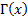is defined as: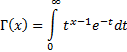The gamma function can be thought of as the extension of the factorial to the entire real (or complex) number set. For non-negative integers it is merely the familiar factorial function,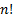, but offset by 1, i.e.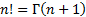. Like factorials, it satisfies the following recurrence relationship: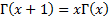The Nematrian website approximates the gamma function using a so-called Lanczos approximation, see also Press et al. (2007), Toth (2004) or Wikipedia: Lanczos approximation.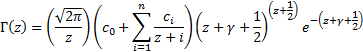The particular Lanczos approximation the Nematrian website uses involves: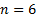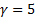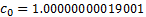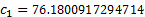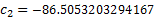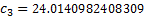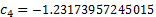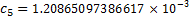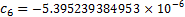For largethere is a risk of overflow, which can be mitigated by using the MnLogGamma function, defined as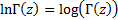.

The Lanczos approximation is valid for arguments in the right complex half-plane, but can be extended to the entire complex plane (where the function is not singular) using the reflection formula, i.e.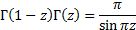Contents | Prev | Next